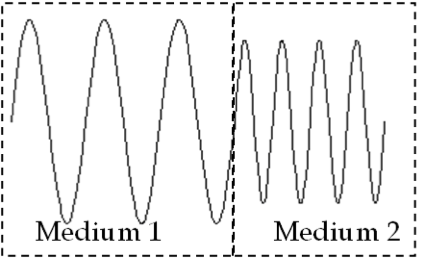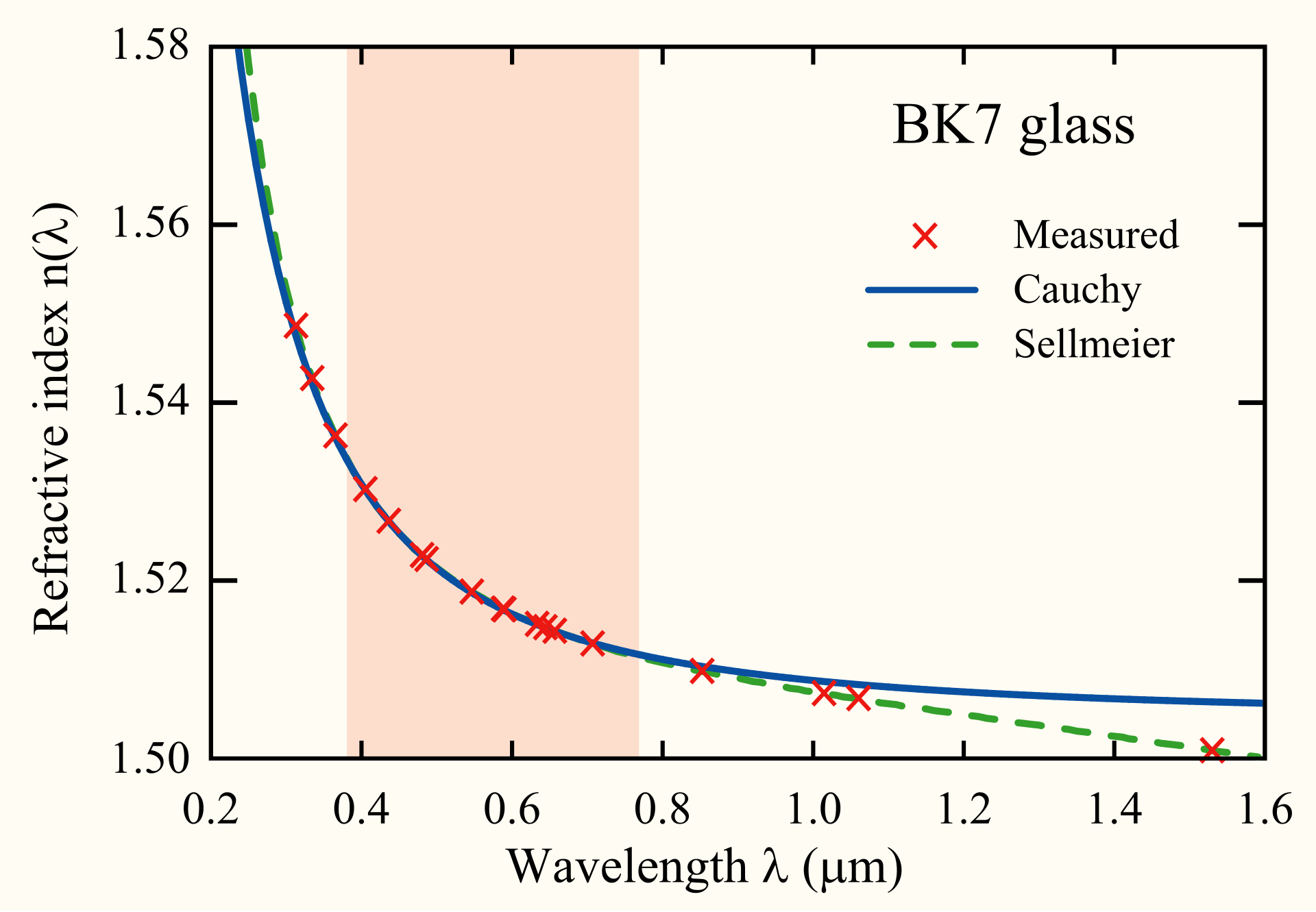## Section46.6Optical Media

When light interacts with a material it can be absorbed, reflected, scattered or transmitted. If a significant part of light is transmitted such that you can see the source of light through the medium, we say that matrial is a transparent medium. An example is clear glass is tranaparent to visible light.

On the other hand, if light passes through the medium but one cannot see the source clearly, the medium is called translucent. In a translucent medium, light scatters around and takes a cicuitous path. For instance, light through mily water.

A medium is called opaque if light cannot pass through it at all. For instance, a piece of metal is opaque to visible light.

It is difficult to label a medium absolutely transparent or opaque, since the transparency depends on the wavelength of light. There are some media, such as glass and lucite, that are transparent to the wavelengths of the visible light to a large extent, and they are often considered transparent enough for optical devices.

### Subsection46.6.1Transparent Medium

In this book you will be studying mainly light through transparent media. therefore, it is important to note down some salient aspects of behavior of light waves in transparent media.

First thing to note is that speed of light varies among transparent media due to their differing dielectric constant. From Maxwell's theory of electricity and magnetism, one can show that sped of light in a linear medium is related to its electric susceptibiliy $\epsilon$ and magnetic permeability $\mu$ by

\begin{equation} v = \frac{1}{\sqrt{\mu\epsilon}}.\tag{46.6.1} \end{equation}

Magnetic permeability of dielectric media are very close to the value in vacuum, $\mu \approx \mu_0\text{.}$ We write electric susceptibility in terms of dielectric constant $\epsilon_r$ and electric susceptibility of vacuum, $\epsilon_0\text{.}$

\begin{equation*} \epsilon = \epsilon_0 \epsilon_r. \end{equation*}

Therefore, speed in light in a linear medium is

\begin{equation} v = \frac{1}{\sqrt{\mu_0\epsilon_0\epsilon_r}} = \frac{c}{\sqrt{\epsilon_r}},\tag{46.6.2} \end{equation}

where $c$ is the speed of light in vacuum.

\begin{equation} c = \frac{1}{\sqrt{\mu_0\epsilon_0}}\tag{46.6.3} \end{equation}

Since dielectric constant is greater than $1\text{,}$ we see that light has lower speed in a material than in vacuum. We also identify $\sqrt{\epsilon_r}$ with the refractive index $n$ of the medium.

\begin{equation} n = \sqrt{\epsilon_r}.\tag{46.6.4} \end{equation}

Therefore, we can write the speed in a medium also in terms of refractive index as

\begin{equation} v = \frac{c}{n}.\label{eq-refractive-index-speed-relation}\tag{46.6.5} \end{equation}

We can use $v=f\lambda$ to replace $v$ and $c=f\lambda_0$ for light of same frequency but different wavelength in vacuum to obtain the following useful relation.

\begin{equation} \lambda = \frac{\lambda_0}{n}.\label{eq-wavelength-in-medium-from-wavelength-in-vacuum}\tag{46.6.6} \end{equation}

Table 46.6.1 gives refractive indices of some common materials for a yellow light.

When a light some frequency $f$ is incident from one medium into another, the frequency of the transmitted light will be same as that of the incident light since it is depedent on the vibration at the original soource of light. But, the wavelength of the transmitted light will be different than that of the incident light since speeds will be different and wavelength $\lambda = v/f\text{.}$ This is illustrated in Figure 46.6.2. If the wavelength is $\lambda_1$ in the medium of refractive index $n_1$ and the same light has a wavelength $\lambda_2$ in the medium of refractive index $n_2\text{,}$ then, using (46.6.6), we get

\begin{equation} n_1 \lambda_1 = n_2 \lambda_2.\tag{46.6.7} \end{equation}Figure 46.6.2. Different wavelengths in different media for the same light. Here, dielectric constant and refractive index of the second medium is larger than that of the first medium. The amplitude is shown smaller in the second medium to show that some of the incident light is reflected at the interface. The reflected wave is not shown here.

### Subsection46.6.2Dispersion of Media

The refractive index $n\text{,}$ or equivalently, the dielectric constant $\epsilon_r\text{,}$ is often a function of wavelength $\lambda$ of light. For instance, the refractive index of flint glass for red light is 1.62 while for a violet light it is 1.67. This difference is responsible for the separation of colors when sun light passes through a prism. The dependence of refractive index on frequency is called dispersion of light.

Figure 46.6.3 shows refractive index of BK7 glass and fitting of the experimental data by two models - the Cauchy equation and Sellmeir equation. Both of these try to fit the experimental curve by using parameters. Cauchy is much simpler fitting model.

\begin{equation} n(\lambda) = A + \frac{B}{\lambda} + \frac{C}{\lambda^2} + \cdots,\tag{46.6.8} \end{equation}

Sellmeir is built on this model. Often a three-parameter Sellmeir equation fits data over a wide range quite well.

\begin{equation} n^2(\lambda) = A + \frac{B\lambda^2}{\lambda^2 - C}.\tag{46.6.9} \end{equation}Figure 46.6.3. Refractive index of BK7 glass as a function of wavelength of light. The experimental data are marked with $\times$ and fits to the data are to Cauchy's eqution (blue) and Sellmeier equation (green). By Bob Mellish at https://en.wikipedia.org/wiki/User:DrBob.

Find speed and wavelength of light in glass if its wavelength in vacuum is $632.8\text{ nm}\text{.}$ Use $n = 1.55$ for the refractive index of glass for the given light.

Hint

Use $v = c/n$ and $f=c/\lambda_0=v/\lambda\text{.}$

$1.94\times 10^8\ \text{m/s}\text{,}$ $408.3\ \text{nm}\text{.}$

Solution

We can find the value of speed easily by dividing the speed in vacuum by the refractive index.

\begin{align*} v \amp = \frac{c}{n} = \frac{3\times 10^8\ \text{m/s}}{1.55} = 1.94\times 10^8\ \text{m/s}. \end{align*}

For the wavelength we note that frequency of light does not change when it passes from one medium into another. Let $\lambda_0$ be the wavelength of the light in vacuum and $\lambda$ be the wavelength of the same light in the medium. The, we will have

\begin{equation*} f = \frac{c}{\lambda_0} = \frac{v}{\lambda}. \end{equation*}

Since $v = c/n\text{,}$ we obtain

\begin{equation*} \lambda = \frac{\lambda_0}{n} = \frac{632.8\ \text{nm}}{1.55} = 408.3\ \text{nm}. \end{equation*}